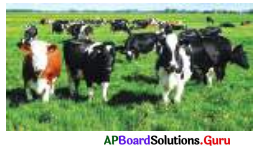# AP Board 7th Class Maths Solutions Chapter 7 Ratio and Proportion Ex 7.1

SCERT AP 7th Class Maths Solutions Pdf Chapter 7 Ratio and Proportion Ex 7.1 Textbook Exercise Questions and Answers.

## AP State Syllabus 7th Class Maths Solutions 7th Lesson Ratio and Proportion Ex 7.1

Question 1.
Pavan and Roshan started a business with ₹ 1,50,000 and ₹ 2,00,000 respectively. After nine months Roshan left the business. At the end of the year, they got a profit of ₹ 45,000. Then find the profits shared by Pavan and Roshafi.
Given Pavan’s investment =₹ 150000
Pavan’s period in business = 1 year = 12 months
Roshan’s investment = ₹ 200000
Rohan’s period in business = 9 months

The ratio of investments of Pavan to Roshan = 150000 : 200000 = 3 : 4
The ratio of periods of business of Pavan to Roshan = 12 : 9 = 4 : 3
The profit should be distributed on the basis of the compound ratio of investments and period = 3 : 4 and 4 : 3 is
3 × 4 : 4 × 3 = 12 : 12

Therefore, compound Ratio =1:1 (i.e.,) they should share profit equally. Profit = ₹ 45,000
Total parts = 1 + 1 = 2
Pavan’s profit = 45000 × $$\frac{1}{2}$$ = ₹ 22500
Roshan’s profit = 45000 – 22500 = ₹ 22,500Question 2.
Salman started a hotel with an amount of ₹ 75,000. After 5 months Deepak joined with an amount of ₹ 80,000. At the end of the year they earned a profit of ₹ 73,000. How will they share their profit?
Given Salman’s investment = ₹ 75000
Salman’s period in business = 1 year = 12 months
Deepak’s investment = ₹ 80000 Deepak’s period in business = 7 months
The ratio of investments of Salman to Deepak = 75000 : 80000 = 15 : 16
The ratio of periods of business of Salman to Deepak = 12 : 7

The profit should be distributed on the basis of compound ratio of 15 : 16 and 12 : 7 is
15 × 12 : 16 × 7 = 180 : 112
= 45 : 28
Therefore, compound ratio = 45 : 28
Profit = ₹ 73,000
Total parts = 45 + 28 = 73

∴ Salman’s profit = 73000 × $$\frac{45}{73}$$
= ₹ 45000

Deepak’s profit = 73000 × $$\frac{28}{73}$$
= ₹ 28,000

Question 3.
Ramayya took a grass field for rent for grazing his 24 cows. After 5 months, Somayya also joined with him for his 40 cows. At the end year they paid a rent of ₹ 35,500. What would be the rent paid by each of them?Given number of cows grazed by Ramayya = 24
Grazed months 1 year =12 months.
Number of cows grazed by Somayya = 40
Grazed months = 7 months.

The ratio number of grazed cows
= 24 : 40 = 3 : 5

The ratio of months grazed = 12 : 7
The rent should be distributed on the basis of compound ratio of 3 : 5 and 12 : 7 is
3 × 12 : 5 × 7 = 36 : 35

Therefore, compound ratio = 36 : 35
Annual Rent = ₹ 35,500
Total parts = 36 + 35 = 71

Rent paid by Rarnavya = 35,500 × $$\frac{36}{71}$$
= ₹ 18,000
Rent paid by Somaa = 35,500 × $$\frac{35}{71}$$
= ₹ 17,500Question 4.
Ravi started a business with ₹ 2,10,000. After a few months, Prakash joined the business with an amount of ₹ 3,60,000. At the end of the year if they got a profit of ₹ 1,20,000 each, then find after how many months did Prakash join in the business?
Given Ravi’s investment = ₹ 2,10,000
Ravi’s period in business = 1 year = 12 months
Prakash’s investment = ₹ 3,60,000

Let, Prakash’s period in business = x months
The Ratio of investments of Ravi and Prakash = 210000 : 360000 = 7 : 12
The ratio of periods of business of Ravi to Prakash = 12 : x

The profit should distributed on the basis of compound ratio of 7 : 12 and 12 : x is
7 × 12 : 12 × x = 84 : 12x
= 7 : x
Therefore, compound ratio = 7 : x
They got equal profits.

So, Total profit = 120000 + 120000 = ₹ 2,40,000
Total parts = 7 + x
So, x = 7
So Prakash joined after (12 – 7) = 5 months
Prakash’s period in business = x
= 7 months
So, Prakash joined in business after (12 – 7) = 5 months.